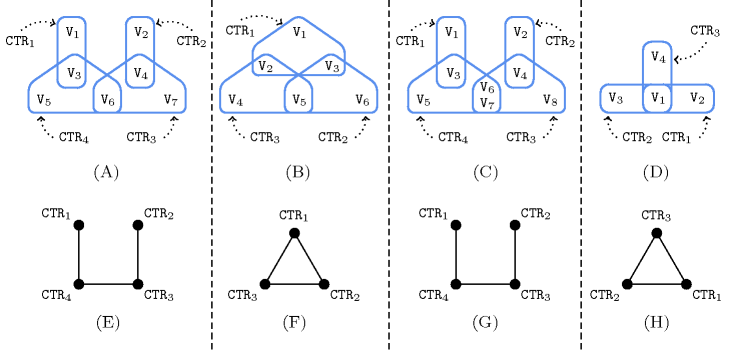### 3.7.29. Berge-acyclic constraint network

A constraint for which the decomposition associated with its usually counter-free deterministic automatonAll the above constraints, except $\mathrm{𝚊𝚖𝚘𝚗𝚐}$, $\mathrm{𝚌𝚑𝚊𝚗𝚐𝚎}$, and $\mathrm{𝚜𝚖𝚘𝚘𝚝𝚑}$ have a deterministic counter-free automaton. The $\mathrm{𝚊𝚖𝚘𝚗𝚐}$ constraint has an automaton involving one counter and a single state, see Figure 5.23.3, while the $\mathrm{𝚌𝚑𝚊𝚗𝚐𝚎}$ and the $\mathrm{𝚜𝚖𝚘𝚘𝚝𝚑}$ constraints have a counter-free non deterministic automaton, see Figures 5.61.5 and 5.355.4. is Berge-acyclic. Arc-consistency for a Berge-acyclic constraint network is achieved by making each constraint of the corresponding network arc-consistent [BeeriFaginMaierYannakakis83]. A constraint network for which the corresponding intersection graph does not contain any cycle and such that, for any pair of constraints, the two sets of involved variables share at most one variable is Berge-acyclic, where Berge-acyclic is defined by the following two conditions:

1. There is no more than one shared variable between any pair of constraints,

2. The hypergraph corresponding to the constraint network does not contain any cycle. Within [Berge87] a cycle of an hypergraph $H$ is defined as “Let $H$ be an hypergraph on a finite set $X$. A cycle of length $k$ ($k\ge 2$) is a sequence $\left({x}_{1},{E}_{1},{x}_{2},{E}_{2},{x}_{3},\cdots ,{E}_{k},{x}_{1}\right)$ such that (1) ${E}_{1},{E}_{2},\cdots ,{E}_{k}$ are distinct edges of $H$, (2) ${x}_{1},{x}_{2},\cdots ,{x}_{k}$ are distinct vertices of $H$, (3) ${x}_{i},{x}_{i+1}\in {E}_{i}$ ($i=1,2,\cdots ,k-1$), (4) ${x}_{k},{x}_{1}\in {E}_{k}$.

The intersection graph of a constraint network is built in the following way: to each vertex corresponds a constraint and there is an edge between two vertices if and only if the sets of variables involved in the two corresponding constraints intersect.

##### Figure 3.7.7. (A) and (D): Berge-acyclic constraint networks; (B) and (C): non Berge-acyclic constraint networks; (E), (F), (G), (H): corresponding intersection graphs.Parts (A), (B), (C) and (D) of Figure 3.7.7 provide four examples of constraint networks, while parts (E), (F), (G) and (F) give their corresponding intersection graphs.

1. The constraint network corresponding to part (A) is Berge-acyclic since its corresponding intersection graph (E) does not contain any cycle and since there is no more than one shared variable between any pair of constraints.

2. The constraint network corresponding to part (B) is not Berge-acyclic since its hypergraph (B) contains a cycle.

3. The constraint network corresponding to (C) is also not Berge-acyclic since its third and fourth constraints share more than one variable.

4. Finally, the constraint network corresponding to (D) is Berge acyclic, even though its intersection graph (H) has a cycle, since its hypergraph (D) does not contain any cycle and since there is no more than one shared variable between any pair of constraints.

If we execute the filtering algorithm of each constraint of a Berge-acyclic constraint network $𝒩$ in an appropriate order then each constraint needs only to be waken twice in order to reach the fix-point. A static ordering for waking the constraints of $𝒩$ can be determined as follows:

• Consider the intersection graph ${𝒢}_{𝒩}$ associated with the constraint network $𝒩$. We perform a topological sort on ${𝒢}_{𝒩}$, which always first selects in the remaining part of ${𝒢}_{𝒩}$ a vertex (i.e., a constraint) which has only a single neighbour. Let ${C}_{1},{C}_{2},\cdots ,{C}_{n}$ be the constraints successively removed by the topological sort.

• Then, the static ordering for reaching a fix-point is given by the sequence ${C}_{1},{C}_{2},\cdots ,{C}_{n-1},{C}_{n},{C}_{n-1},\cdots ,{C}_{2},{C}_{1}$, where each constraint is woken at most twice. This can be done by using the notion of propagator group [LagerkvistSchulte09]. This facility allows the user of a solver controlling the order of execution of a group of constraints. Propagator groups are useful, both to guaranty the theoretical worst case complexity of a decomposition, and for accelerating convergence to the fix-point in practice.

If we consider the Berge-acyclic constraint network given by Part (D) of Figure 3.7.7 an appropriate order for waking the constraints could for instance be ${\mathrm{CTR}}_{1}$, ${\mathrm{CTR}}_{4}$, ${\mathrm{CTR}}_{2}$, ${\mathrm{CTR}}_{3}$, ${\mathrm{CTR}}_{2}$, ${\mathrm{CTR}}_{4}$, ${\mathrm{CTR}}_{1}$.

For heuristics that try creating a Berge-acyclic constraint network see also the keyword heuristics and Berge-acyclic constraint network.